Скачать презентацию Data Mining Concepts and Techniques 3 rd ed

0eb864c81b8c2dd5182fb0fe155f26f4.ppt

• Количество слайдов: 52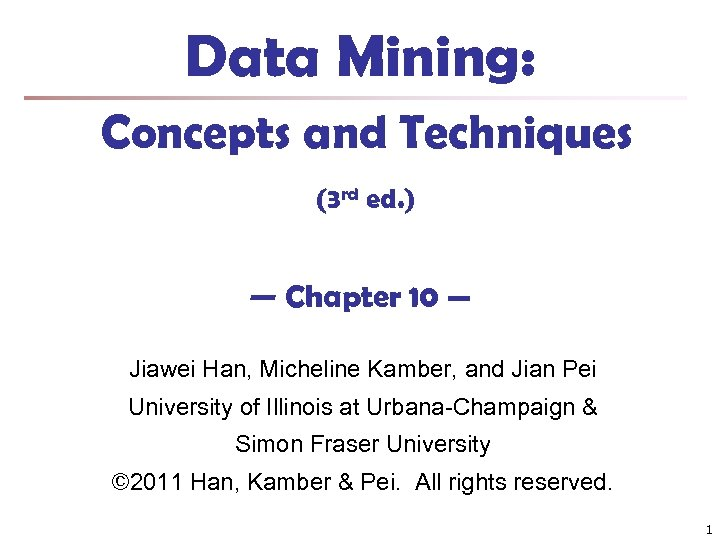Data Mining: Concepts and Techniques (3 rd ed. ) — Chapter 10 — Jiawei Han, Micheline Kamber, and Jian Pei University of Illinois at Urbana-Champaign & Simon Fraser University © 2011 Han, Kamber & Pei. All rights reserved. 1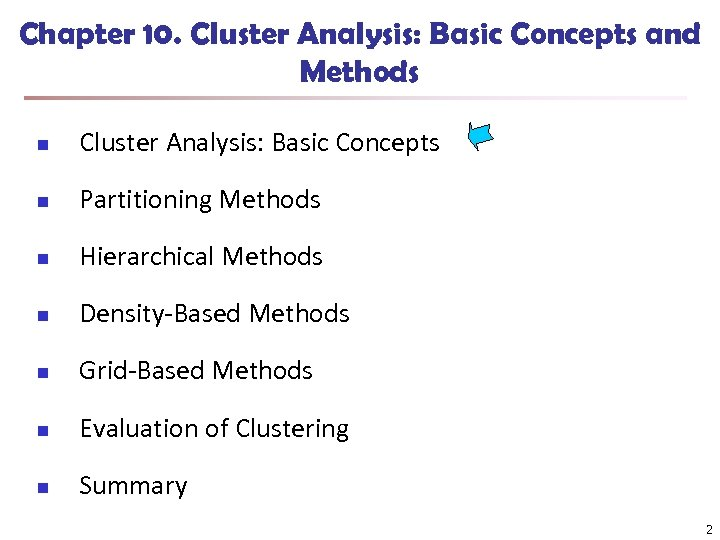Chapter 10. Cluster Analysis: Basic Concepts and Methods n Cluster Analysis: Basic Concepts n Partitioning Methods n Hierarchical Methods n Density-Based Methods n Grid-Based Methods n Evaluation of Clustering n Summary 2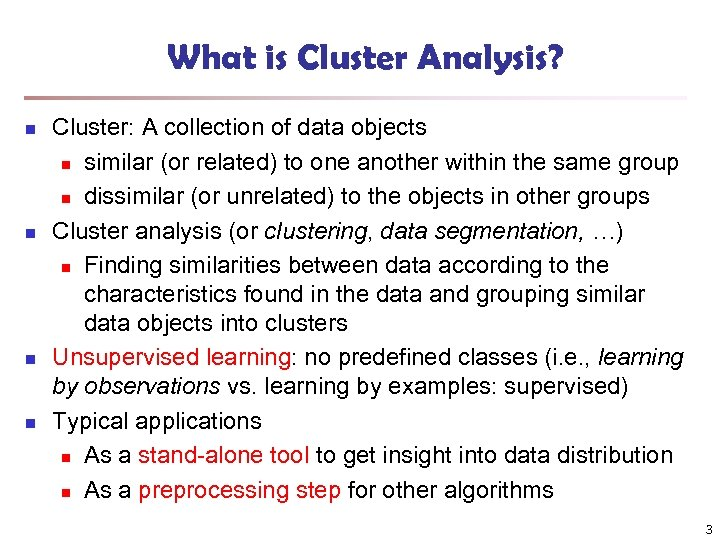What is Cluster Analysis? n n Cluster: A collection of data objects n similar (or related) to one another within the same group n dissimilar (or unrelated) to the objects in other groups Cluster analysis (or clustering, data segmentation, …) n Finding similarities between data according to the characteristics found in the data and grouping similar data objects into clusters Unsupervised learning: no predefined classes (i. e. , learning by observations vs. learning by examples: supervised) Typical applications n As a stand-alone tool to get insight into data distribution n As a preprocessing step for other algorithms 3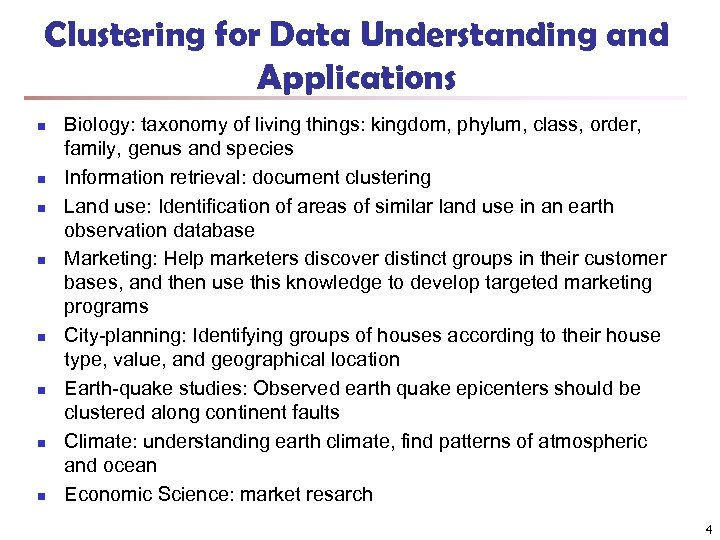Clustering for Data Understanding and Applications n n n n Biology: taxonomy of living things: kingdom, phylum, class, order, family, genus and species Information retrieval: document clustering Land use: Identification of areas of similar land use in an earth observation database Marketing: Help marketers discover distinct groups in their customer bases, and then use this knowledge to develop targeted marketing programs City-planning: Identifying groups of houses according to their house type, value, and geographical location Earth-quake studies: Observed earth quake epicenters should be clustered along continent faults Climate: understanding earth climate, find patterns of atmospheric and ocean Economic Science: market resarch 4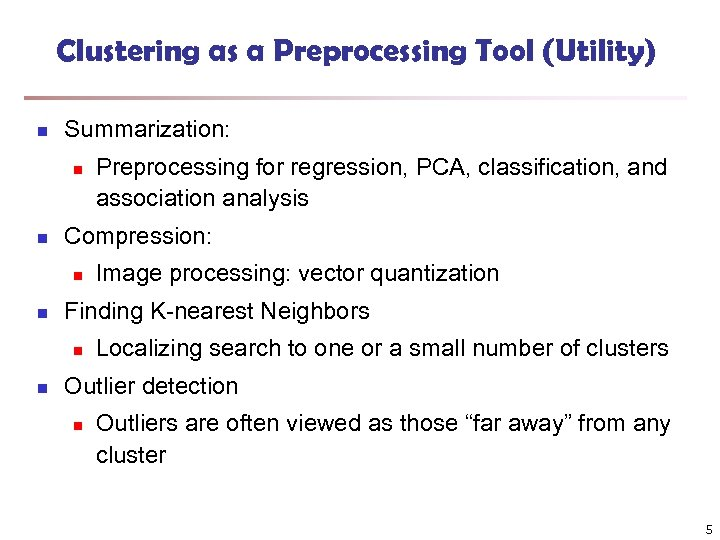Clustering as a Preprocessing Tool (Utility) n Summarization: n n Compression: n n Image processing: vector quantization Finding K-nearest Neighbors n n Preprocessing for regression, PCA, classification, and association analysis Localizing search to one or a small number of clusters Outlier detection n Outliers are often viewed as those “far away” from any cluster 5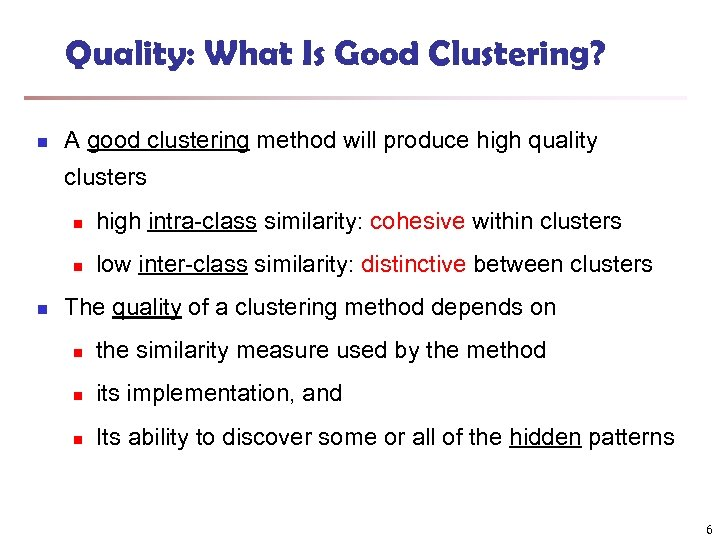Quality: What Is Good Clustering? n A good clustering method will produce high quality clusters n n n high intra-class similarity: cohesive within clusters low inter-class similarity: distinctive between clusters The quality of a clustering method depends on n the similarity measure used by the method n its implementation, and n Its ability to discover some or all of the hidden patterns 6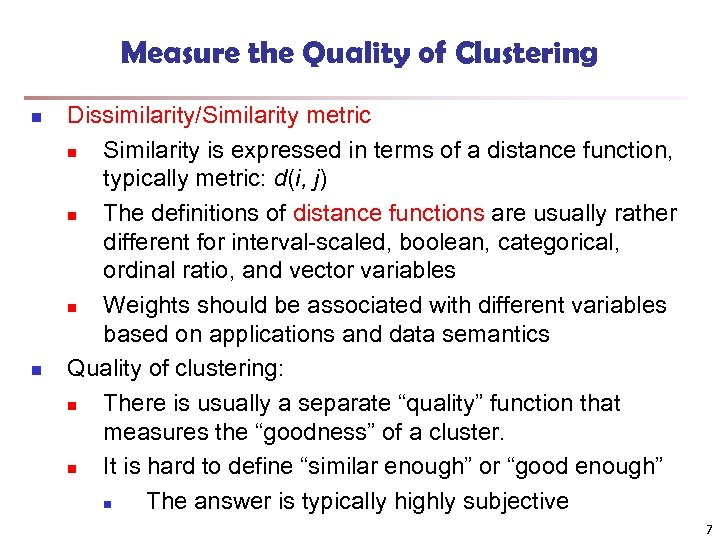Measure the Quality of Clustering n n Dissimilarity/Similarity metric n Similarity is expressed in terms of a distance function, typically metric: d(i, j) n The definitions of distance functions are usually rather different for interval-scaled, boolean, categorical, ordinal ratio, and vector variables n Weights should be associated with different variables based on applications and data semantics Quality of clustering: n There is usually a separate “quality” function that measures the “goodness” of a cluster. n It is hard to define “similar enough” or “good enough” n The answer is typically highly subjective 7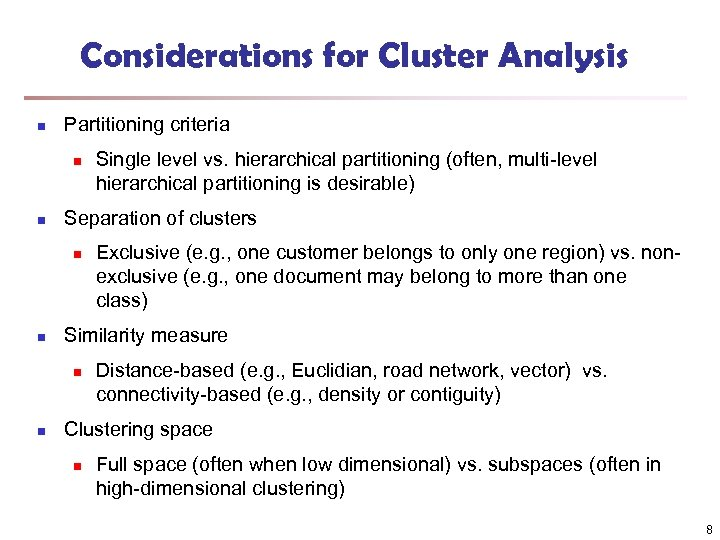Considerations for Cluster Analysis n Partitioning criteria n n Separation of clusters n n Exclusive (e. g. , one customer belongs to only one region) vs. nonexclusive (e. g. , one document may belong to more than one class) Similarity measure n n Single level vs. hierarchical partitioning (often, multi-level hierarchical partitioning is desirable) Distance-based (e. g. , Euclidian, road network, vector) vs. connectivity-based (e. g. , density or contiguity) Clustering space n Full space (often when low dimensional) vs. subspaces (often in high-dimensional clustering) 8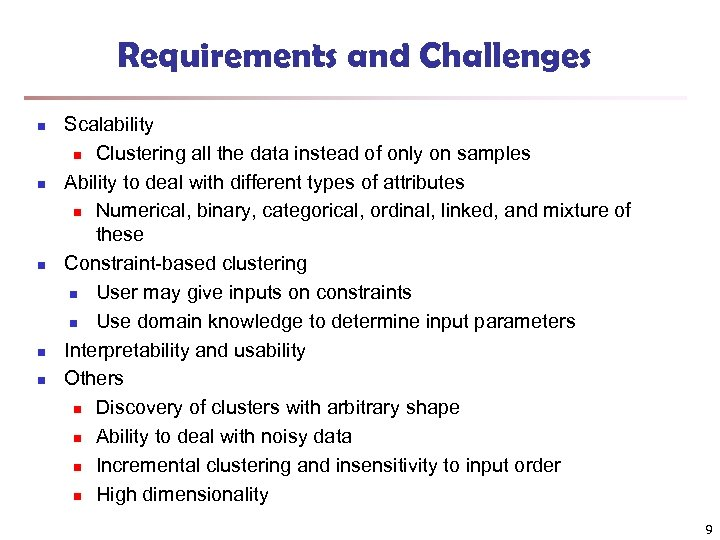Requirements and Challenges n n n Scalability n Clustering all the data instead of only on samples Ability to deal with different types of attributes n Numerical, binary, categorical, ordinal, linked, and mixture of these Constraint-based clustering n User may give inputs on constraints n Use domain knowledge to determine input parameters Interpretability and usability Others n Discovery of clusters with arbitrary shape n Ability to deal with noisy data n Incremental clustering and insensitivity to input order n High dimensionality 9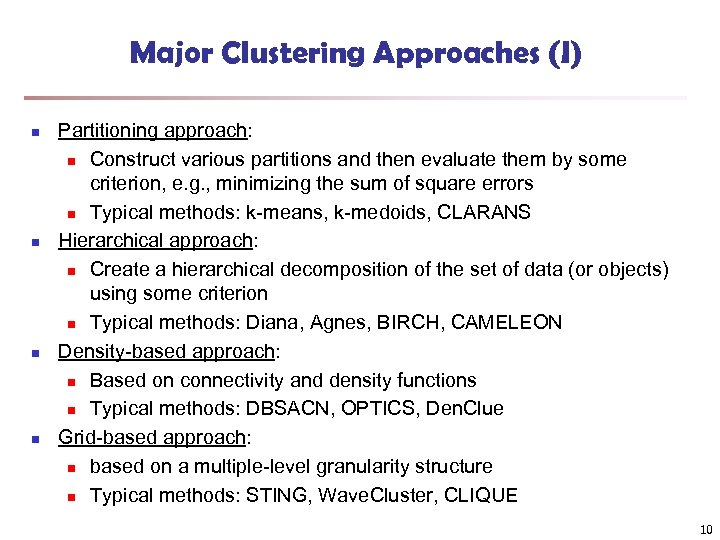Major Clustering Approaches (I) n n Partitioning approach: n Construct various partitions and then evaluate them by some criterion, e. g. , minimizing the sum of square errors n Typical methods: k-means, k-medoids, CLARANS Hierarchical approach: n Create a hierarchical decomposition of the set of data (or objects) using some criterion n Typical methods: Diana, Agnes, BIRCH, CAMELEON Density-based approach: n Based on connectivity and density functions n Typical methods: DBSACN, OPTICS, Den. Clue Grid-based approach: n based on a multiple-level granularity structure n Typical methods: STING, Wave. Cluster, CLIQUE 10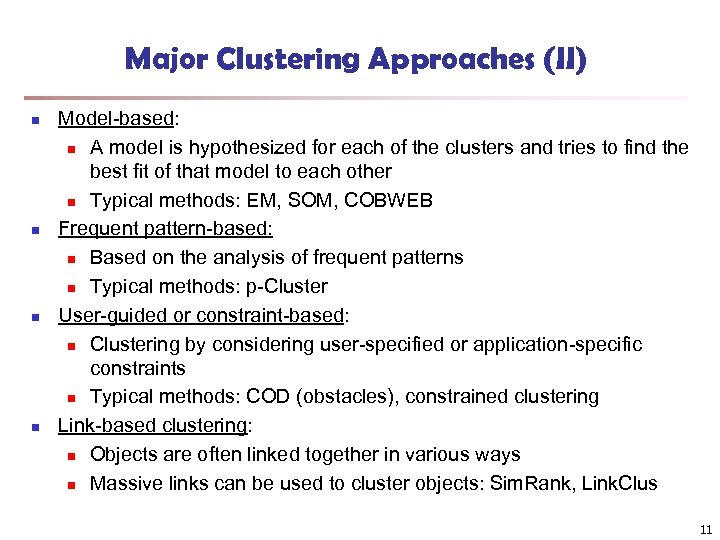Major Clustering Approaches (II) n n Model-based: n A model is hypothesized for each of the clusters and tries to find the best fit of that model to each other n Typical methods: EM, SOM, COBWEB Frequent pattern-based: n Based on the analysis of frequent patterns n Typical methods: p-Cluster User-guided or constraint-based: n Clustering by considering user-specified or application-specific constraints n Typical methods: COD (obstacles), constrained clustering Link-based clustering: n Objects are often linked together in various ways n Massive links can be used to cluster objects: Sim. Rank, Link. Clus 11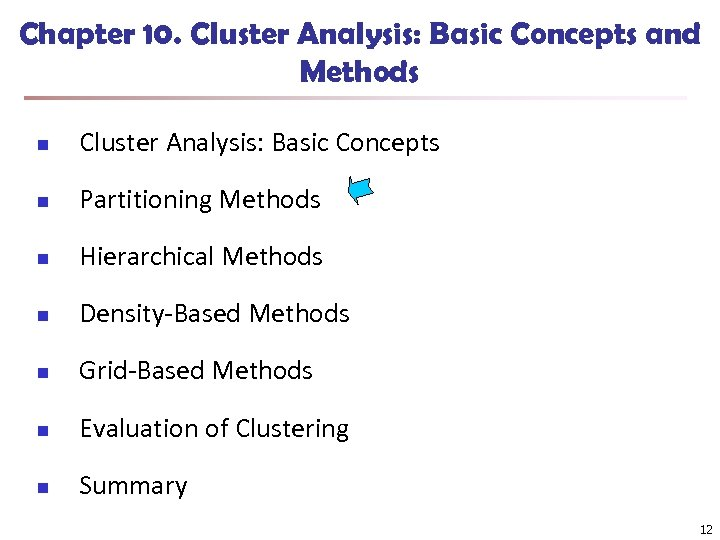Chapter 10. Cluster Analysis: Basic Concepts and Methods n Cluster Analysis: Basic Concepts n Partitioning Methods n Hierarchical Methods n Density-Based Methods n Grid-Based Methods n Evaluation of Clustering n Summary 12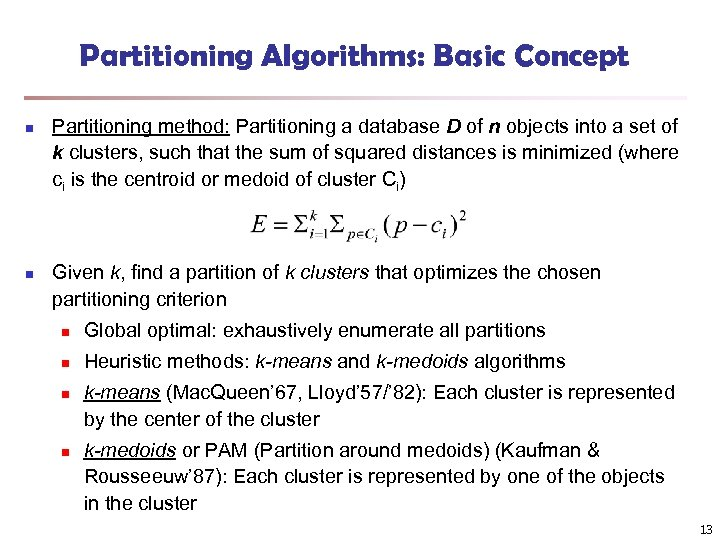Partitioning Algorithms: Basic Concept n n Partitioning method: Partitioning a database D of n objects into a set of k clusters, such that the sum of squared distances is minimized (where ci is the centroid or medoid of cluster Ci) Given k, find a partition of k clusters that optimizes the chosen partitioning criterion n Global optimal: exhaustively enumerate all partitions n Heuristic methods: k-means and k-medoids algorithms n n k-means (Mac. Queen’ 67, Lloyd’ 57/’ 82): Each cluster is represented by the center of the cluster k-medoids or PAM (Partition around medoids) (Kaufman & Rousseeuw’ 87): Each cluster is represented by one of the objects in the cluster 13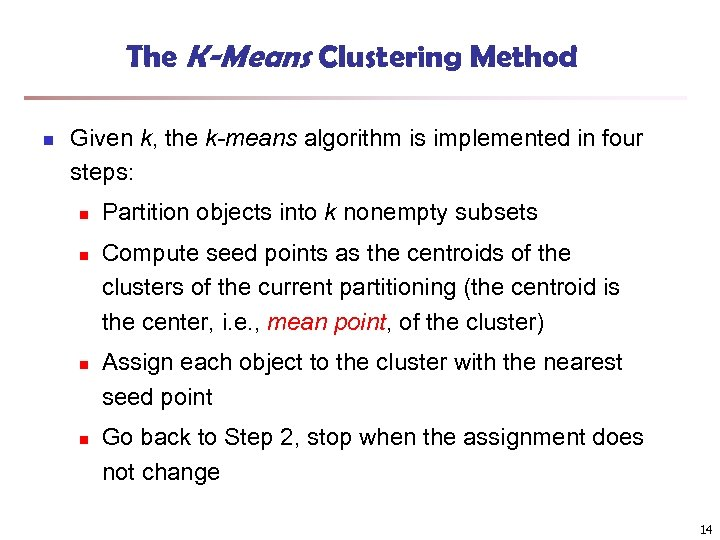The K-Means Clustering Method n Given k, the k-means algorithm is implemented in four steps: n n Partition objects into k nonempty subsets Compute seed points as the centroids of the clusters of the current partitioning (the centroid is the center, i. e. , mean point, of the cluster) Assign each object to the cluster with the nearest seed point Go back to Step 2, stop when the assignment does not change 14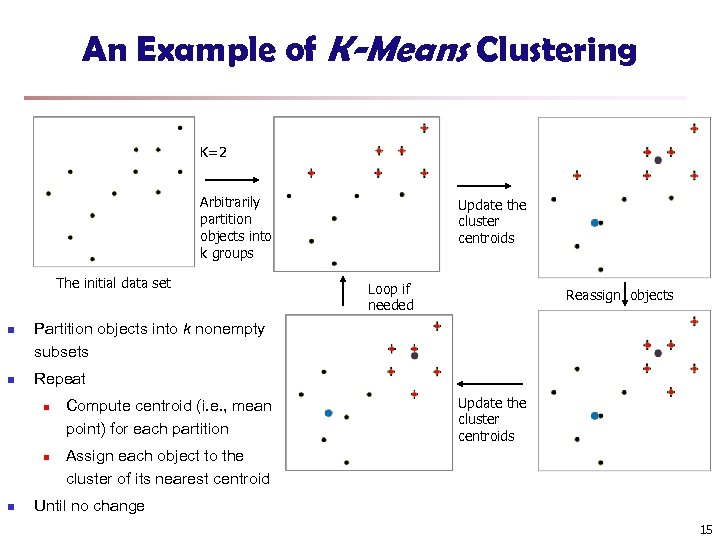An Example of K-Means Clustering K=2 Arbitrarily partition objects into k groups The initial data set n n Loop if needed Reassign objects Partition objects into k nonempty subsets Repeat n n n Update the cluster centroids Compute centroid (i. e. , mean point) for each partition Update the cluster centroids Assign each object to the cluster of its nearest centroid Until no change 15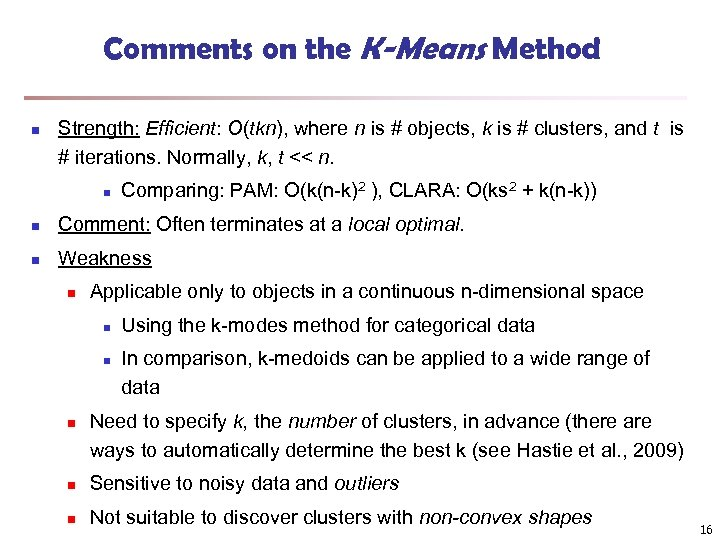Comments on the K-Means Method n Strength: Efficient: O(tkn), where n is # objects, k is # clusters, and t is # iterations. Normally, k, t << n. n Comparing: PAM: O(k(n-k)2 ), CLARA: O(ks 2 + k(n-k)) n Comment: Often terminates at a local optimal. n Weakness n Applicable only to objects in a continuous n-dimensional space n n n Using the k-modes method for categorical data In comparison, k-medoids can be applied to a wide range of data Need to specify k, the number of clusters, in advance (there are ways to automatically determine the best k (see Hastie et al. , 2009) n Sensitive to noisy data and outliers n Not suitable to discover clusters with non-convex shapes 16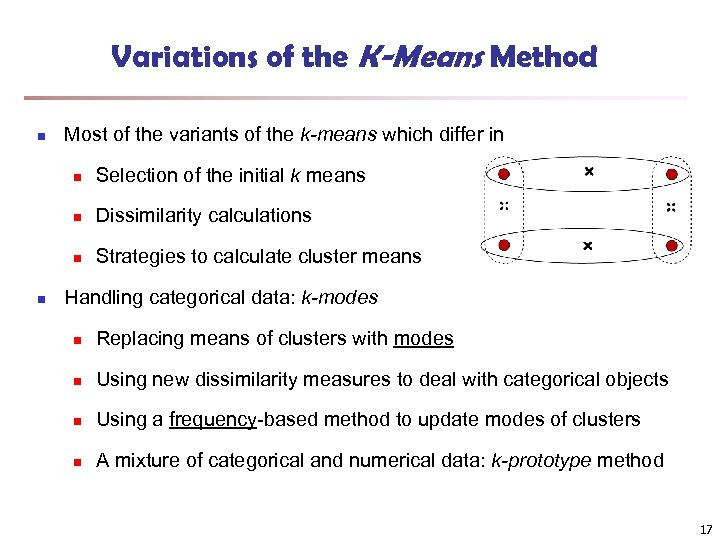Variations of the K-Means Method n Most of the variants of the k-means which differ in n n Dissimilarity calculations n n Selection of the initial k means Strategies to calculate cluster means Handling categorical data: k-modes n Replacing means of clusters with modes n Using new dissimilarity measures to deal with categorical objects n Using a frequency-based method to update modes of clusters n A mixture of categorical and numerical data: k-prototype method 17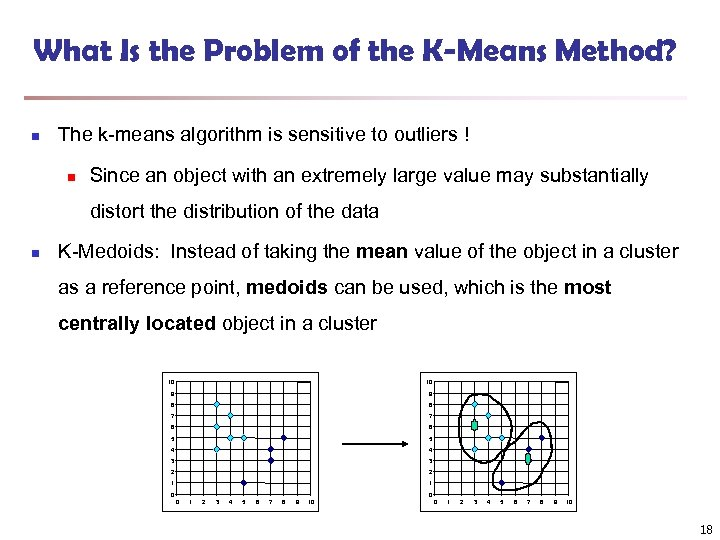What Is the Problem of the K-Means Method? n The k-means algorithm is sensitive to outliers ! n Since an object with an extremely large value may substantially distort the distribution of the data n K-Medoids: Instead of taking the mean value of the object in a cluster as a reference point, medoids can be used, which is the most centrally located object in a cluster 10 10 9 9 8 8 7 7 6 6 5 5 4 4 3 3 2 2 1 1 0 0 0 1 2 3 4 5 6 7 8 9 10 18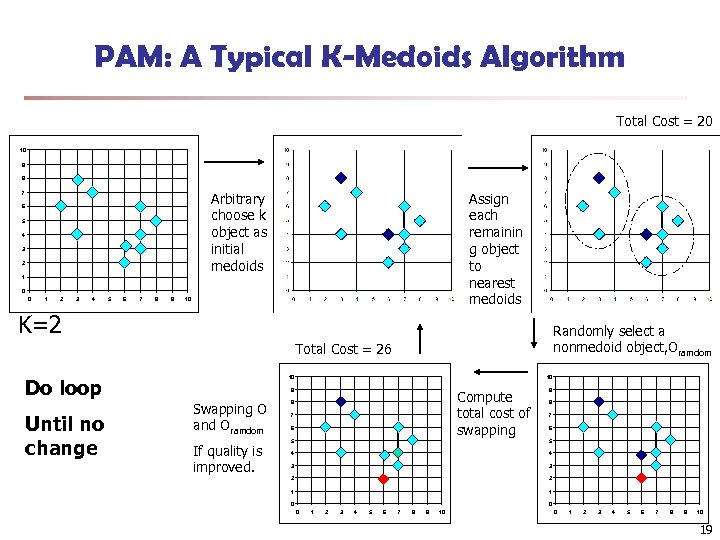PAM: A Typical K-Medoids Algorithm Total Cost = 20 10 9 8 Arbitrary choose k object as initial medoids 7 6 5 4 3 2 Assign each remainin g object to nearest medoids 1 0 0 1 2 3 4 5 6 7 8 9 10 K=2 Randomly select a nonmedoid object, Oramdom Total Cost = 26 Do loop Until no change 10 10 9 Swapping O and Oramdom If quality is improved. Compute total cost of swapping 8 7 6 9 8 7 6 5 5 4 4 3 3 2 2 1 1 0 0 0 1 2 3 4 5 6 7 8 9 10 19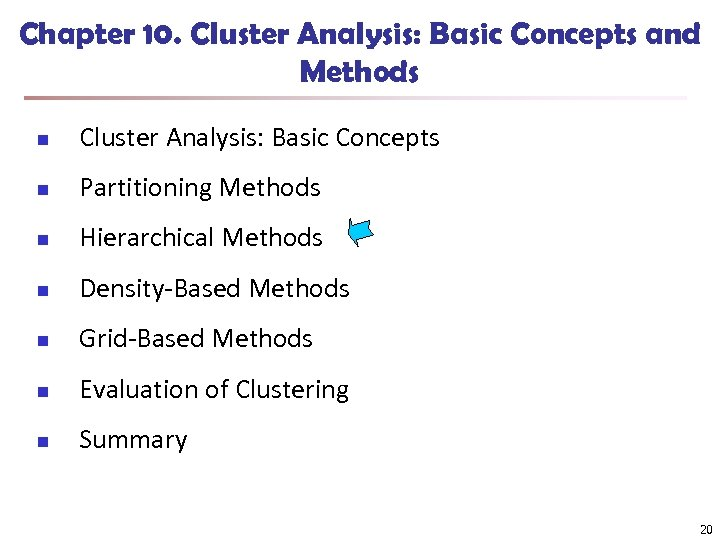Chapter 10. Cluster Analysis: Basic Concepts and Methods n Cluster Analysis: Basic Concepts n Partitioning Methods n Hierarchical Methods n Density-Based Methods n Grid-Based Methods n Evaluation of Clustering n Summary 20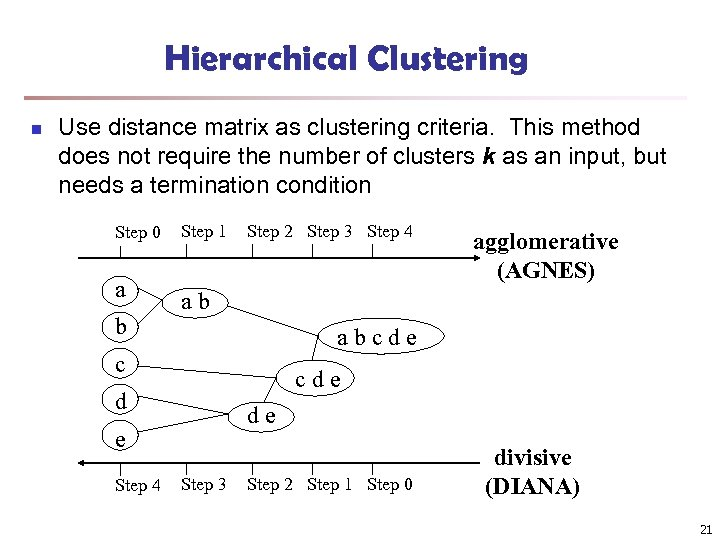Hierarchical Clustering n Use distance matrix as clustering criteria. This method does not require the number of clusters k as an input, but needs a termination condition Step 0 a b Step 1 Step 2 Step 3 Step 4 ab abcde c cde d de e Step 4 agglomerative (AGNES) Step 3 Step 2 Step 1 Step 0 divisive (DIANA) 21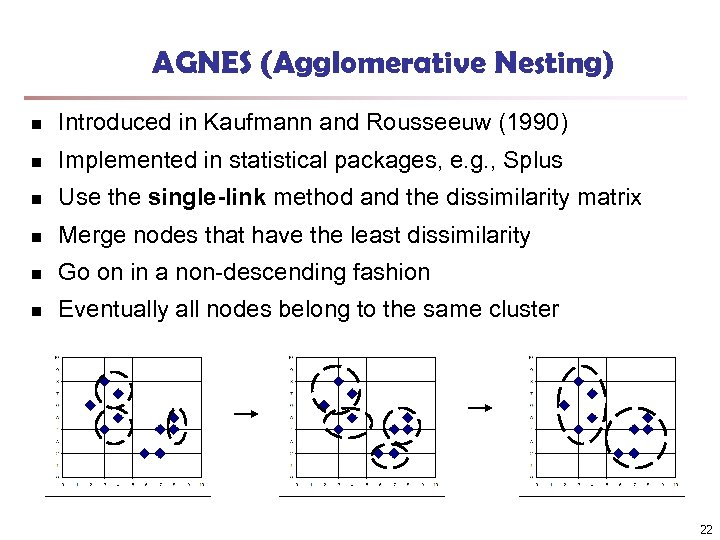AGNES (Agglomerative Nesting) n Introduced in Kaufmann and Rousseeuw (1990) n Implemented in statistical packages, e. g. , Splus n Use the single-link method and the dissimilarity matrix n Merge nodes that have the least dissimilarity n Go on in a non-descending fashion n Eventually all nodes belong to the same cluster 22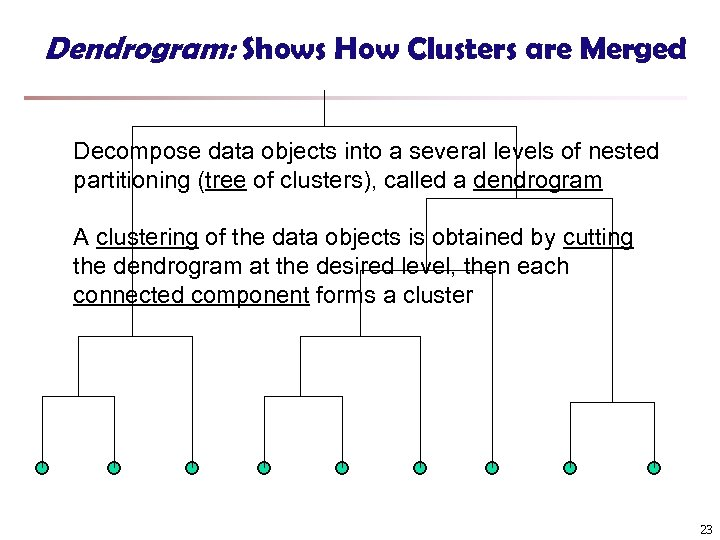Dendrogram: Shows How Clusters are Merged Decompose data objects into a several levels of nested partitioning (tree of clusters), called a dendrogram A clustering of the data objects is obtained by cutting the dendrogram at the desired level, then each connected component forms a cluster 23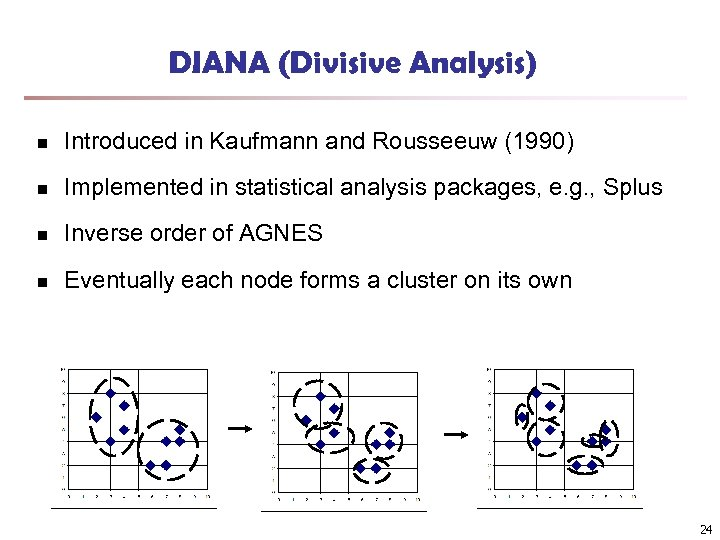DIANA (Divisive Analysis) n Introduced in Kaufmann and Rousseeuw (1990) n Implemented in statistical analysis packages, e. g. , Splus n Inverse order of AGNES n Eventually each node forms a cluster on its own 24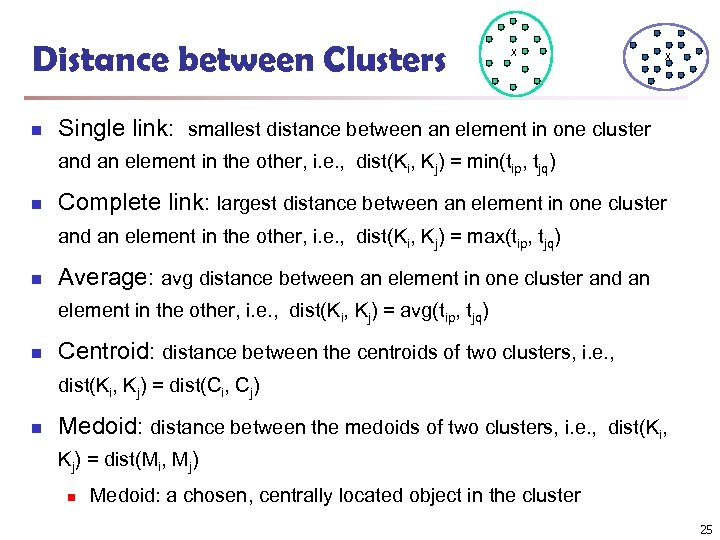Distance between Clusters n X X Single link: smallest distance between an element in one cluster and an element in the other, i. e. , dist(Ki, Kj) = min(tip, tjq) n Complete link: largest distance between an element in one cluster and an element in the other, i. e. , dist(Ki, Kj) = max(tip, tjq) n Average: avg distance between an element in one cluster and an element in the other, i. e. , dist(Ki, Kj) = avg(tip, tjq) n Centroid: distance between the centroids of two clusters, i. e. , dist(Ki, Kj) = dist(Ci, Cj) n Medoid: distance between the medoids of two clusters, i. e. , dist(Ki, Kj) = dist(Mi, Mj) n Medoid: a chosen, centrally located object in the cluster 25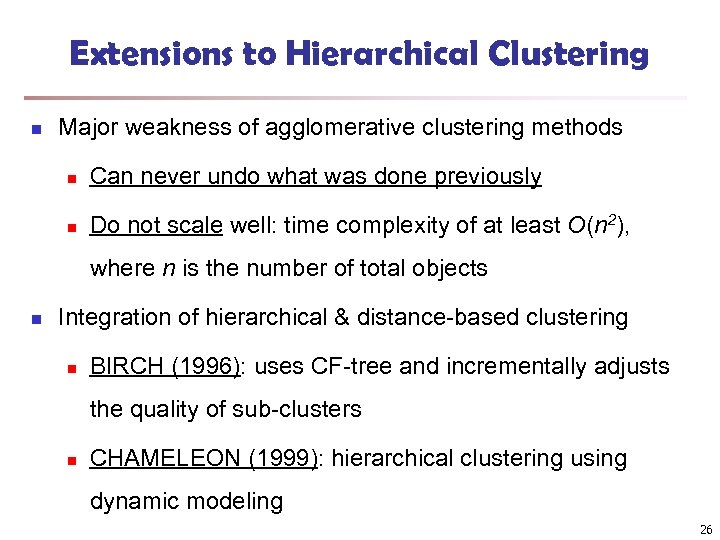Extensions to Hierarchical Clustering n Major weakness of agglomerative clustering methods n Can never undo what was done previously n Do not scale well: time complexity of at least O(n 2), where n is the number of total objects n Integration of hierarchical & distance-based clustering n BIRCH (1996): uses CF-tree and incrementally adjusts the quality of sub-clusters n CHAMELEON (1999): hierarchical clustering using dynamic modeling 26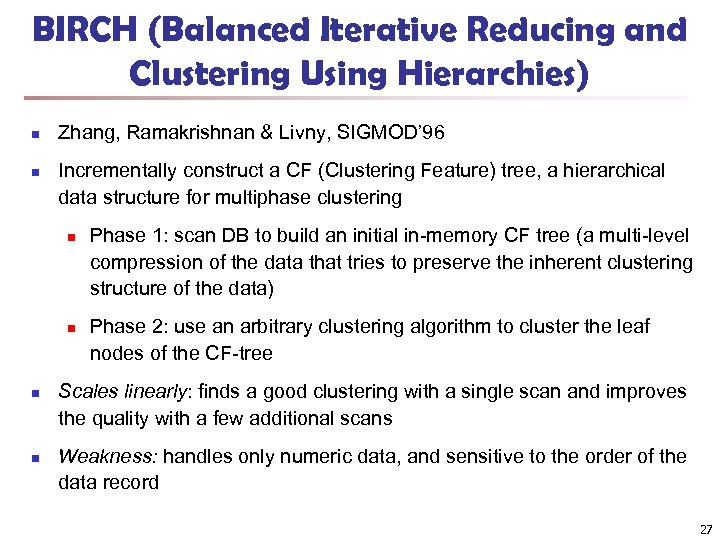BIRCH (Balanced Iterative Reducing and Clustering Using Hierarchies) n n Zhang, Ramakrishnan & Livny, SIGMOD’ 96 Incrementally construct a CF (Clustering Feature) tree, a hierarchical data structure for multiphase clustering n n Phase 1: scan DB to build an initial in-memory CF tree (a multi-level compression of the data that tries to preserve the inherent clustering structure of the data) Phase 2: use an arbitrary clustering algorithm to cluster the leaf nodes of the CF-tree Scales linearly: finds a good clustering with a single scan and improves the quality with a few additional scans Weakness: handles only numeric data, and sensitive to the order of the data record 27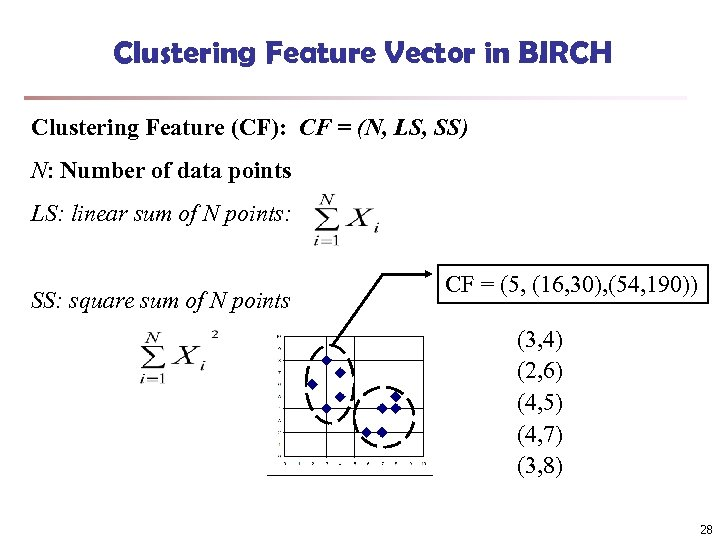Clustering Feature Vector in BIRCH Clustering Feature (CF): CF = (N, LS, SS) N: Number of data points LS: linear sum of N points: SS: square sum of N points CF = (5, (16, 30), (54, 190)) (3, 4) (2, 6) (4, 5) (4, 7) (3, 8) 28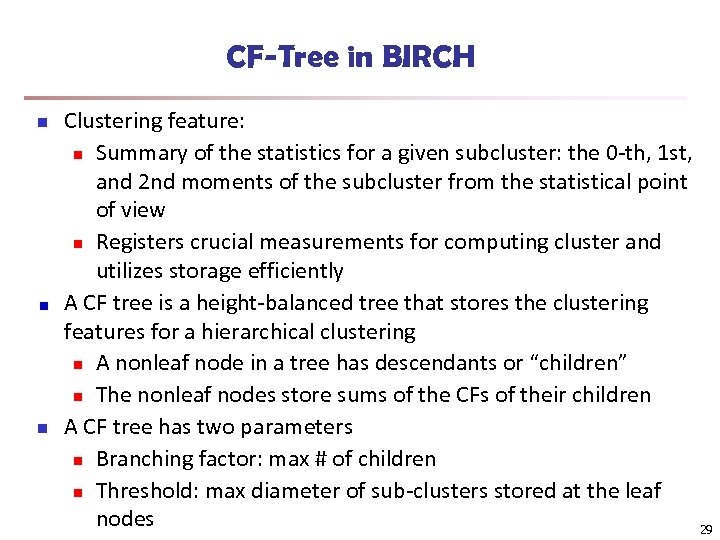CF-Tree in BIRCH n n Clustering feature: n Summary of the statistics for a given subcluster: the 0 -th, 1 st, and 2 nd moments of the subcluster from the statistical point of view n Registers crucial measurements for computing cluster and utilizes storage efficiently A CF tree is a height-balanced tree that stores the clustering features for a hierarchical clustering n A nonleaf node in a tree has descendants or “children” n The nonleaf nodes store sums of the CFs of their children A CF tree has two parameters n Branching factor: max # of children n Threshold: max diameter of sub-clusters stored at the leaf nodes 29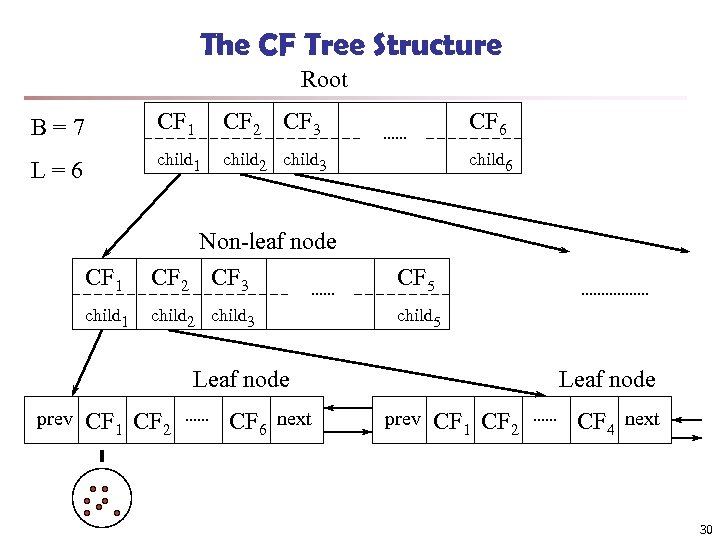The CF Tree Structure Root B=7 CF 1 CF 2 CF 3 CF 6 L=6 child 1 child 2 child 3 child 6 CF 1 Non-leaf node CF 2 CF 3 CF 5 child 1 child 2 child 3 child 5 Leaf node prev CF 1 CF 2 CF 6 next Leaf node prev CF 1 CF 2 CF 4 next 30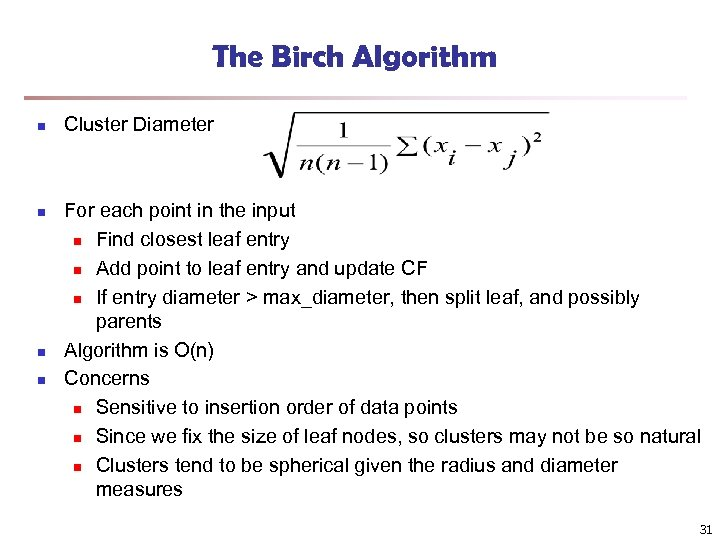The Birch Algorithm n n Cluster Diameter For each point in the input n Find closest leaf entry n Add point to leaf entry and update CF n If entry diameter > max_diameter, then split leaf, and possibly parents Algorithm is O(n) Concerns n Sensitive to insertion order of data points n Since we fix the size of leaf nodes, so clusters may not be so natural n Clusters tend to be spherical given the radius and diameter measures 31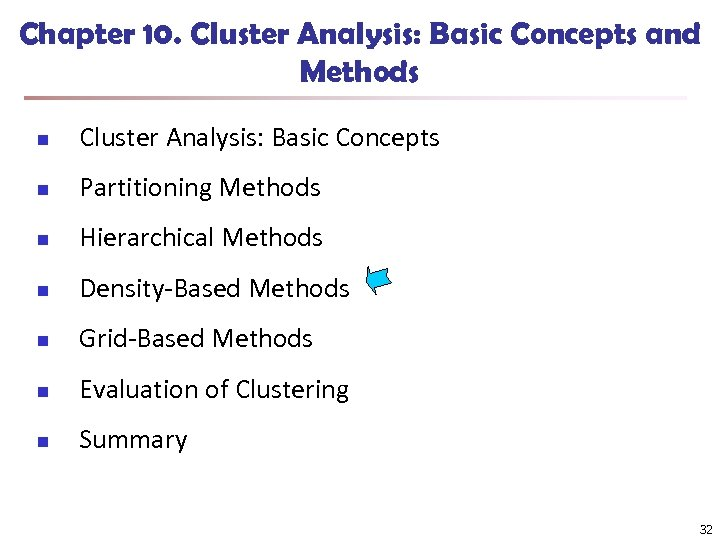Chapter 10. Cluster Analysis: Basic Concepts and Methods n Cluster Analysis: Basic Concepts n Partitioning Methods n Hierarchical Methods n Density-Based Methods n Grid-Based Methods n Evaluation of Clustering n Summary 32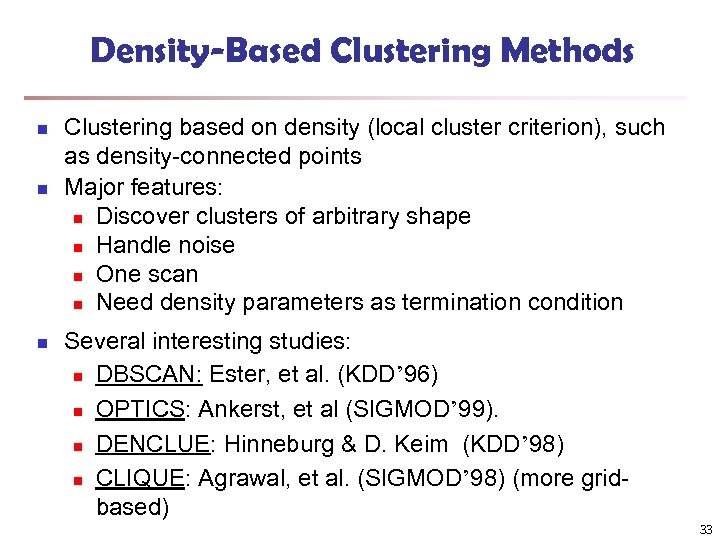Density-Based Clustering Methods n n n Clustering based on density (local cluster criterion), such as density-connected points Major features: n Discover clusters of arbitrary shape n Handle noise n One scan n Need density parameters as termination condition Several interesting studies: n DBSCAN: Ester, et al. (KDD’ 96) n OPTICS: Ankerst, et al (SIGMOD’ 99). n DENCLUE: Hinneburg & D. Keim (KDD’ 98) n CLIQUE: Agrawal, et al. (SIGMOD’ 98) (more gridbased) 33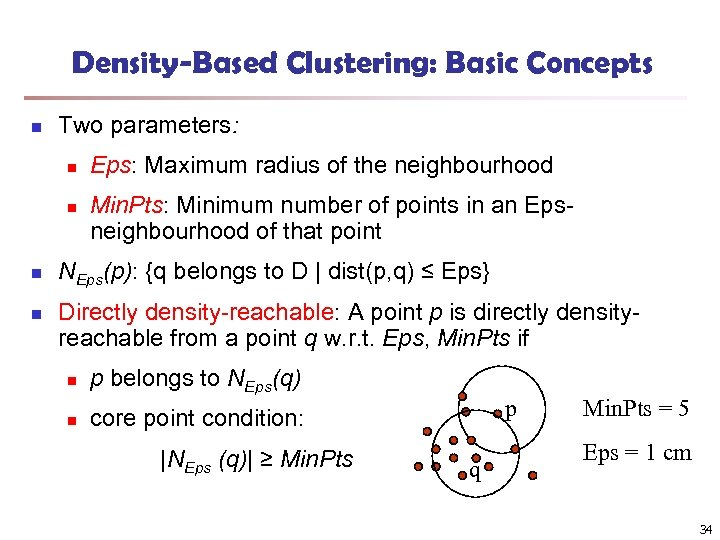Density-Based Clustering: Basic Concepts n Two parameters: n n Eps: Maximum radius of the neighbourhood Min. Pts: Minimum number of points in an Epsneighbourhood of that point NEps(p): {q belongs to D | dist(p, q) ≤ Eps} Directly density-reachable: A point p is directly densityreachable from a point q w. r. t. Eps, Min. Pts if n p belongs to NEps(q) n core point condition: |NEps (q)| ≥ Min. Pts p q Min. Pts = 5 Eps = 1 cm 34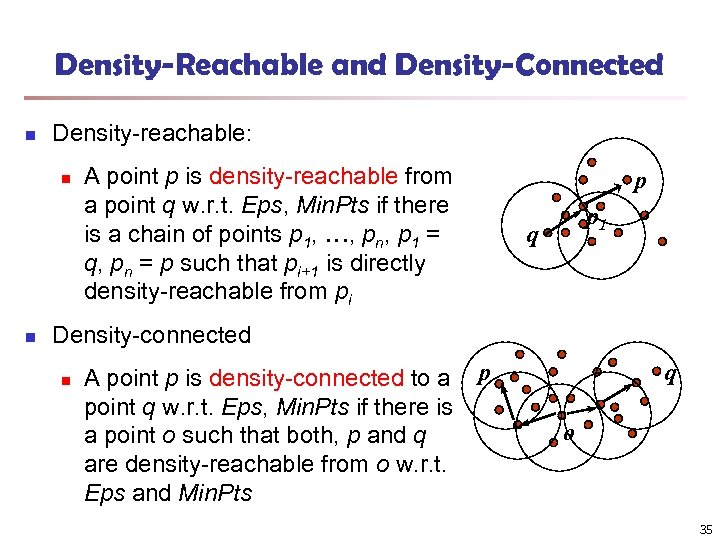Density-Reachable and Density-Connected n Density-reachable: n n A point p is density-reachable from a point q w. r. t. Eps, Min. Pts if there is a chain of points p 1, …, pn, p 1 = q, pn = p such that pi+1 is directly density-reachable from pi p p 1 q Density-connected n A point p is density-connected to a p point q w. r. t. Eps, Min. Pts if there is a point o such that both, p and q are density-reachable from o w. r. t. Eps and Min. Pts q o 35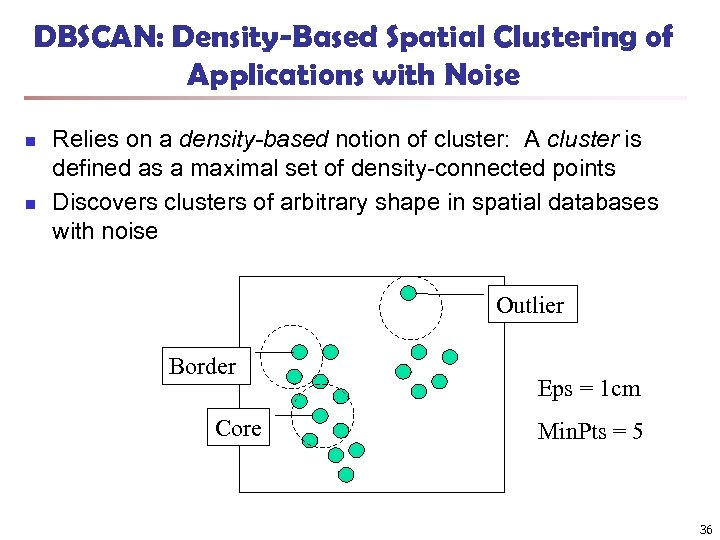DBSCAN: Density-Based Spatial Clustering of Applications with Noise n n Relies on a density-based notion of cluster: A cluster is defined as a maximal set of density-connected points Discovers clusters of arbitrary shape in spatial databases with noise Outlier Border Core Eps = 1 cm Min. Pts = 5 36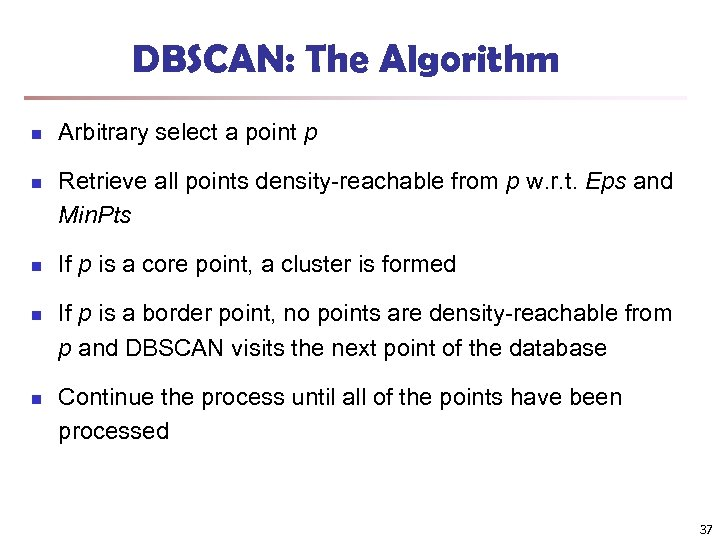DBSCAN: The Algorithm n n n Arbitrary select a point p Retrieve all points density-reachable from p w. r. t. Eps and Min. Pts If p is a core point, a cluster is formed If p is a border point, no points are density-reachable from p and DBSCAN visits the next point of the database Continue the process until all of the points have been processed 37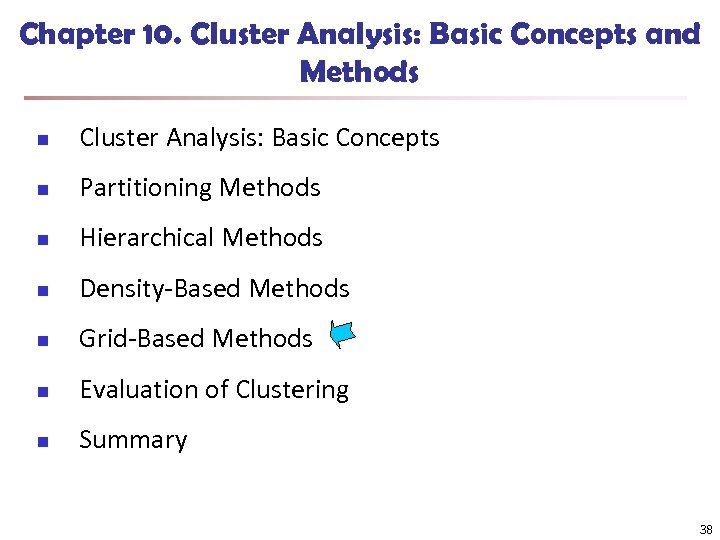Chapter 10. Cluster Analysis: Basic Concepts and Methods n Cluster Analysis: Basic Concepts n Partitioning Methods n Hierarchical Methods n Density-Based Methods n Grid-Based Methods n Evaluation of Clustering n Summary 38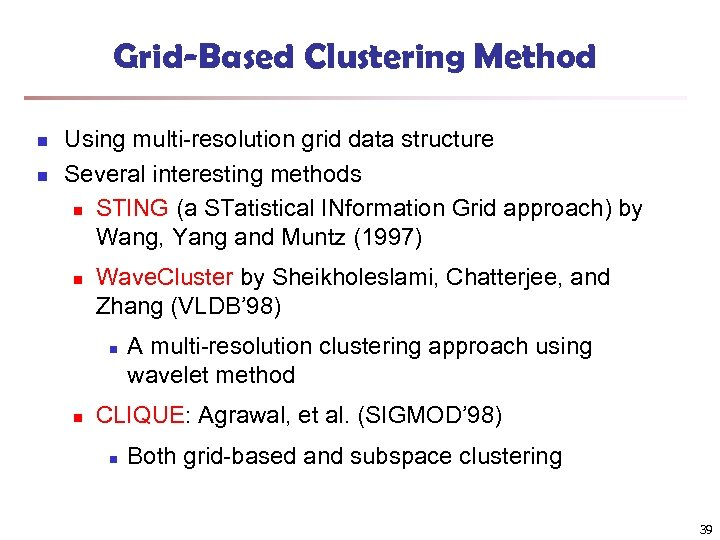Grid-Based Clustering Method n n Using multi-resolution grid data structure Several interesting methods n STING (a STatistical INformation Grid approach) by Wang, Yang and Muntz (1997) n Wave. Cluster by Sheikholeslami, Chatterjee, and Zhang (VLDB’ 98) n n A multi-resolution clustering approach using wavelet method CLIQUE: Agrawal, et al. (SIGMOD’ 98) n Both grid-based and subspace clustering 39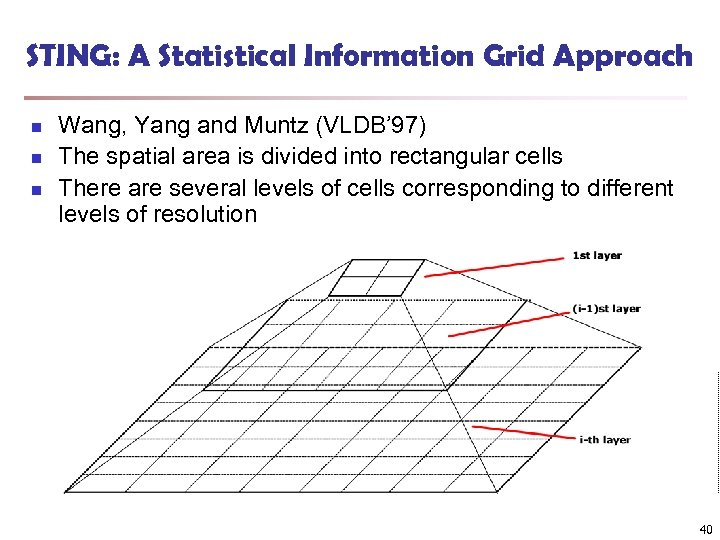STING: A Statistical Information Grid Approach n n n Wang, Yang and Muntz (VLDB’ 97) The spatial area is divided into rectangular cells There are several levels of cells corresponding to different levels of resolution 40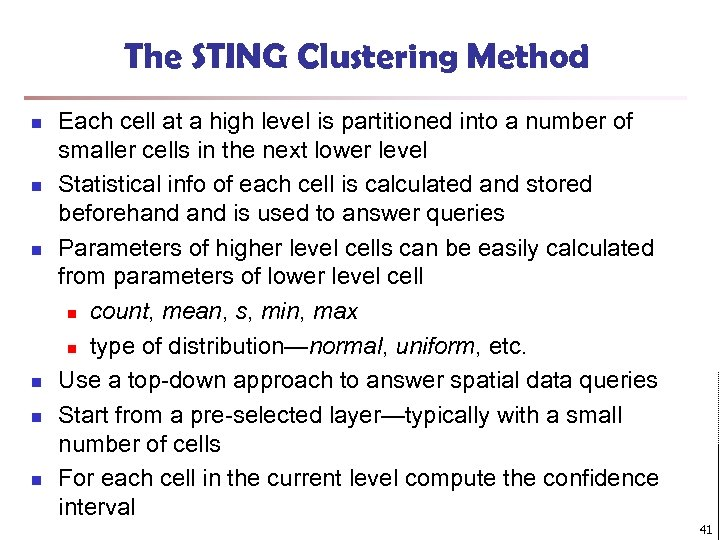The STING Clustering Method n n n Each cell at a high level is partitioned into a number of smaller cells in the next lower level Statistical info of each cell is calculated and stored beforehand is used to answer queries Parameters of higher level cells can be easily calculated from parameters of lower level cell n count, mean, s, min, max n type of distribution—normal, uniform, etc. Use a top-down approach to answer spatial data queries Start from a pre-selected layer—typically with a small number of cells For each cell in the current level compute the confidence interval 41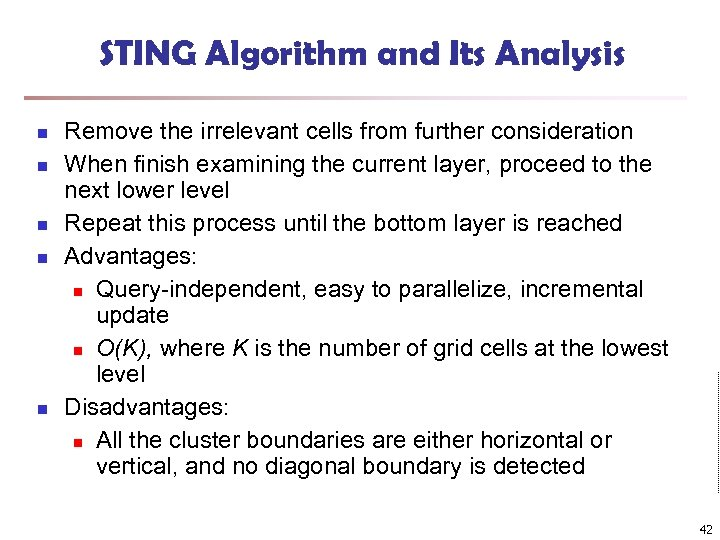STING Algorithm and Its Analysis n n n Remove the irrelevant cells from further consideration When finish examining the current layer, proceed to the next lower level Repeat this process until the bottom layer is reached Advantages: n Query-independent, easy to parallelize, incremental update n O(K), where K is the number of grid cells at the lowest level Disadvantages: n All the cluster boundaries are either horizontal or vertical, and no diagonal boundary is detected 42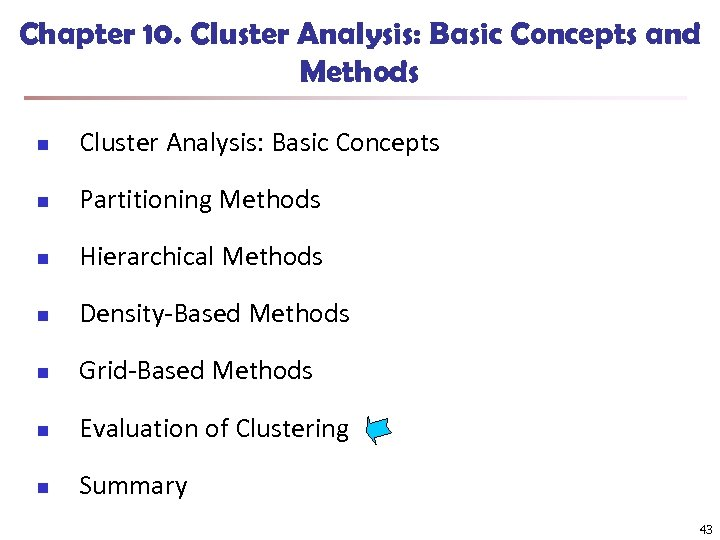Chapter 10. Cluster Analysis: Basic Concepts and Methods n Cluster Analysis: Basic Concepts n Partitioning Methods n Hierarchical Methods n Density-Based Methods n Grid-Based Methods n Evaluation of Clustering n Summary 43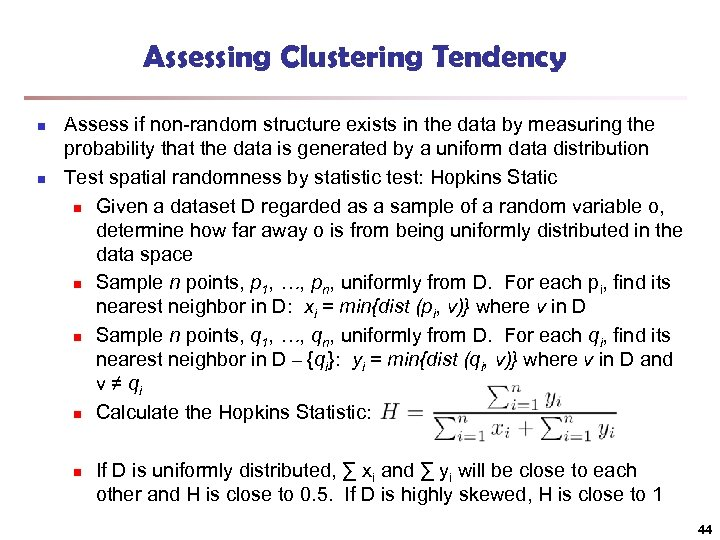Assessing Clustering Tendency n n Assess if non-random structure exists in the data by measuring the probability that the data is generated by a uniform data distribution Test spatial randomness by statistic test: Hopkins Static n Given a dataset D regarded as a sample of a random variable o, determine how far away o is from being uniformly distributed in the data space n Sample n points, p 1, …, pn, uniformly from D. For each pi, find its nearest neighbor in D: xi = min{dist (pi, v)} where v in D n Sample n points, q 1, …, qn, uniformly from D. For each qi, find its nearest neighbor in D – {qi}: yi = min{dist (qi, v)} where v in D and v ≠ qi n Calculate the Hopkins Statistic: n If D is uniformly distributed, ∑ xi and ∑ yi will be close to each other and H is close to 0. 5. If D is highly skewed, H is close to 1 44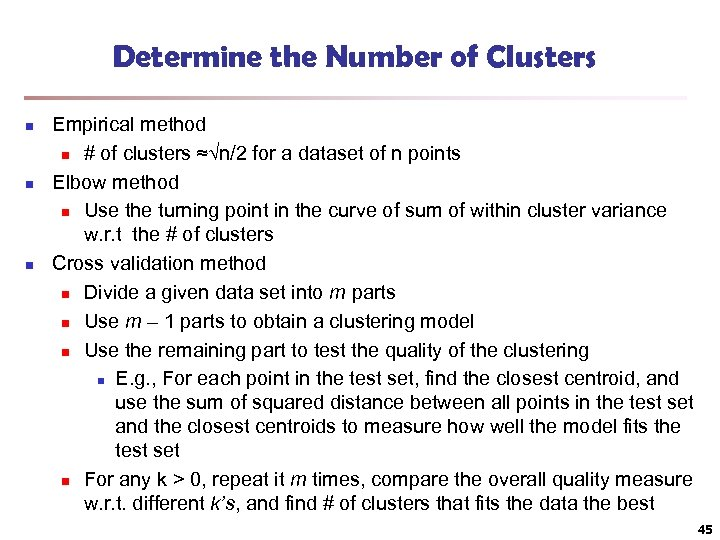Determine the Number of Clusters n n n Empirical method n # of clusters ≈√n/2 for a dataset of n points Elbow method n Use the turning point in the curve of sum of within cluster variance w. r. t the # of clusters Cross validation method n Divide a given data set into m parts n Use m – 1 parts to obtain a clustering model n Use the remaining part to test the quality of the clustering n E. g. , For each point in the test set, find the closest centroid, and use the sum of squared distance between all points in the test set and the closest centroids to measure how well the model fits the test set n For any k > 0, repeat it m times, compare the overall quality measure w. r. t. different k’s, and find # of clusters that fits the data the best 45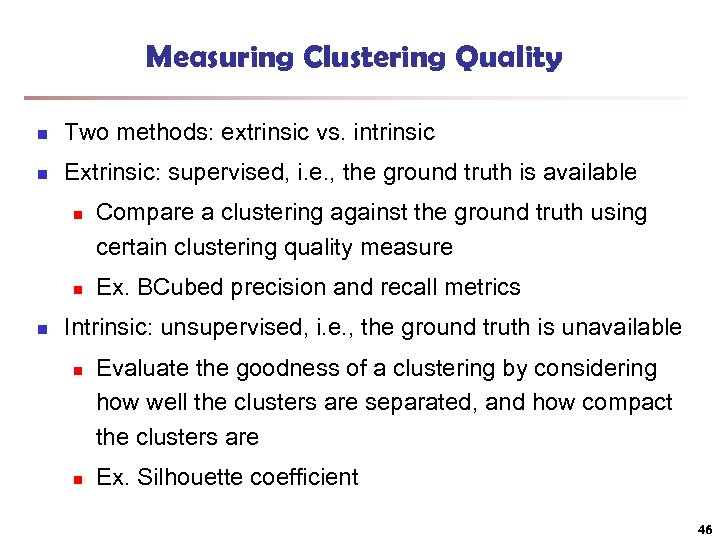Measuring Clustering Quality n Two methods: extrinsic vs. intrinsic n Extrinsic: supervised, i. e. , the ground truth is available n n n Compare a clustering against the ground truth using certain clustering quality measure Ex. BCubed precision and recall metrics Intrinsic: unsupervised, i. e. , the ground truth is unavailable n n Evaluate the goodness of a clustering by considering how well the clusters are separated, and how compact the clusters are Ex. Silhouette coefficient 46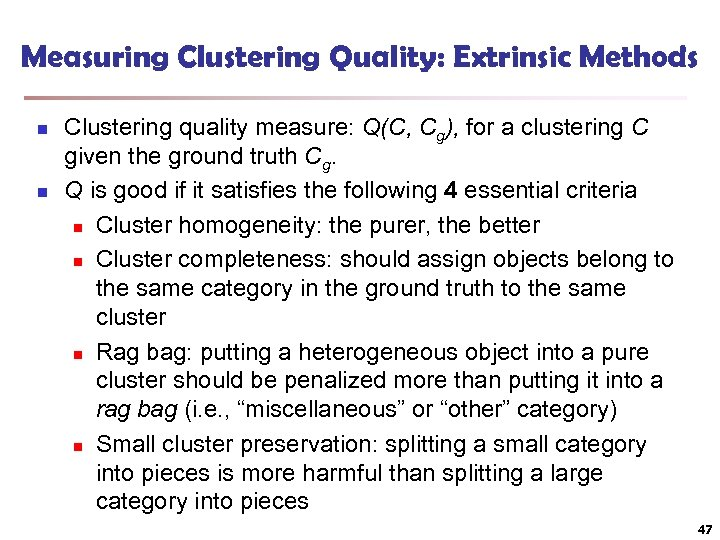Measuring Clustering Quality: Extrinsic Methods n n Clustering quality measure: Q(C, Cg), for a clustering C given the ground truth Cg. Q is good if it satisfies the following 4 essential criteria n Cluster homogeneity: the purer, the better n Cluster completeness: should assign objects belong to the same category in the ground truth to the same cluster n Rag bag: putting a heterogeneous object into a pure cluster should be penalized more than putting it into a rag bag (i. e. , “miscellaneous” or “other” category) n Small cluster preservation: splitting a small category into pieces is more harmful than splitting a large category into pieces 47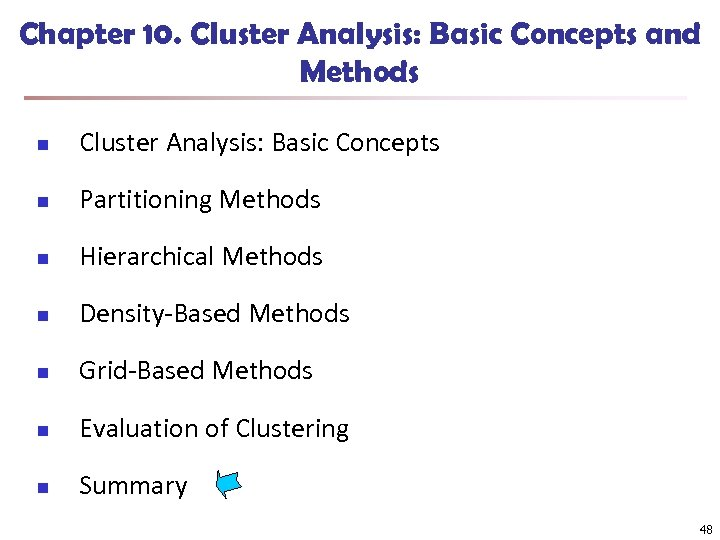Chapter 10. Cluster Analysis: Basic Concepts and Methods n Cluster Analysis: Basic Concepts n Partitioning Methods n Hierarchical Methods n Density-Based Methods n Grid-Based Methods n Evaluation of Clustering n Summary 48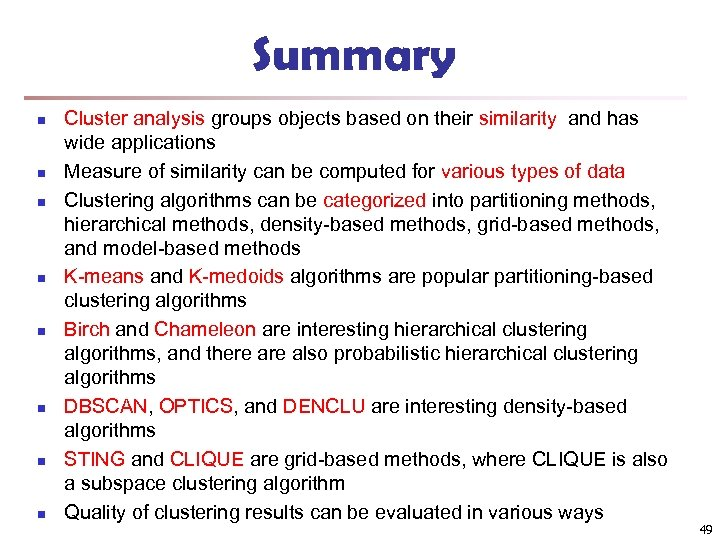Summary n n n n Cluster analysis groups objects based on their similarity and has wide applications Measure of similarity can be computed for various types of data Clustering algorithms can be categorized into partitioning methods, hierarchical methods, density-based methods, grid-based methods, and model-based methods K-means and K-medoids algorithms are popular partitioning-based clustering algorithms Birch and Chameleon are interesting hierarchical clustering algorithms, and there also probabilistic hierarchical clustering algorithms DBSCAN, OPTICS, and DENCLU are interesting density-based algorithms STING and CLIQUE are grid-based methods, where CLIQUE is also a subspace clustering algorithm Quality of clustering results can be evaluated in various ways 49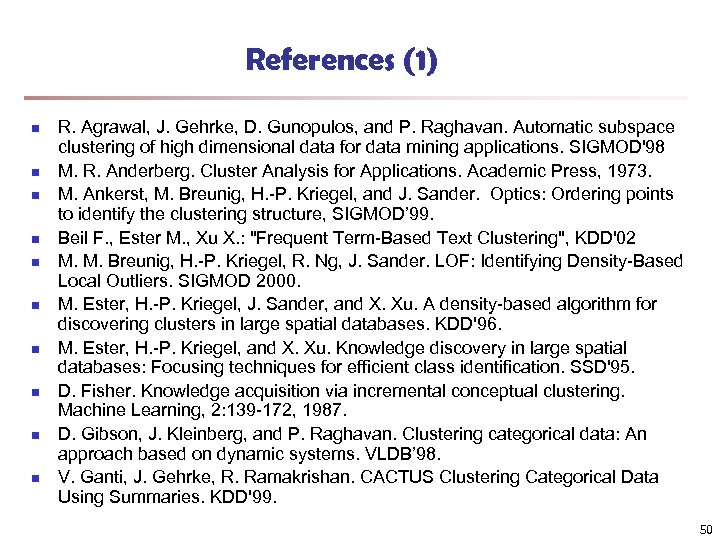References (1) n n n n n R. Agrawal, J. Gehrke, D. Gunopulos, and P. Raghavan. Automatic subspace clustering of high dimensional data for data mining applications. SIGMOD'98 M. R. Anderberg. Cluster Analysis for Applications. Academic Press, 1973. M. Ankerst, M. Breunig, H. -P. Kriegel, and J. Sander. Optics: Ordering points to identify the clustering structure, SIGMOD’ 99. Beil F. , Ester M. , Xu X. : "Frequent Term-Based Text Clustering", KDD'02 M. M. Breunig, H. -P. Kriegel, R. Ng, J. Sander. LOF: Identifying Density-Based Local Outliers. SIGMOD 2000. M. Ester, H. -P. Kriegel, J. Sander, and X. Xu. A density-based algorithm for discovering clusters in large spatial databases. KDD'96. M. Ester, H. -P. Kriegel, and X. Xu. Knowledge discovery in large spatial databases: Focusing techniques for efficient class identification. SSD'95. D. Fisher. Knowledge acquisition via incremental conceptual clustering. Machine Learning, 2: 139 -172, 1987. D. Gibson, J. Kleinberg, and P. Raghavan. Clustering categorical data: An approach based on dynamic systems. VLDB’ 98. V. Ganti, J. Gehrke, R. Ramakrishan. CACTUS Clustering Categorical Data Using Summaries. KDD'99. 50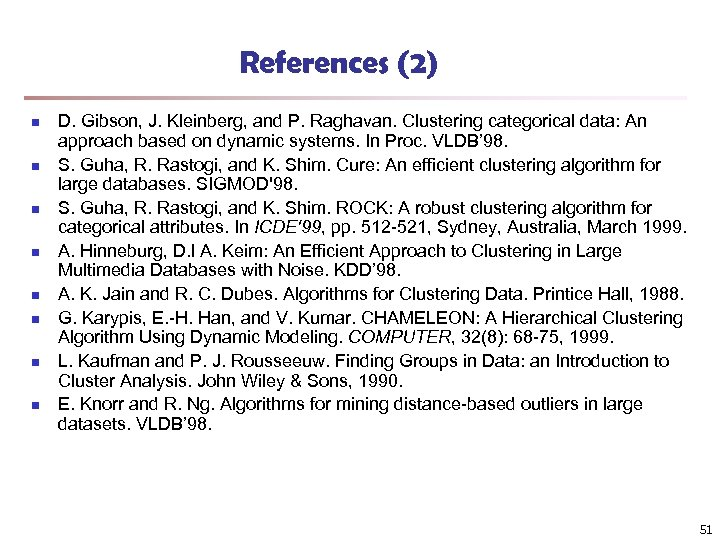References (2) n n n n D. Gibson, J. Kleinberg, and P. Raghavan. Clustering categorical data: An approach based on dynamic systems. In Proc. VLDB’ 98. S. Guha, R. Rastogi, and K. Shim. Cure: An efficient clustering algorithm for large databases. SIGMOD'98. S. Guha, R. Rastogi, and K. Shim. ROCK: A robust clustering algorithm for categorical attributes. In ICDE'99, pp. 512 -521, Sydney, Australia, March 1999. A. Hinneburg, D. l A. Keim: An Efficient Approach to Clustering in Large Multimedia Databases with Noise. KDD’ 98. A. K. Jain and R. C. Dubes. Algorithms for Clustering Data. Printice Hall, 1988. G. Karypis, E. -H. Han, and V. Kumar. CHAMELEON: A Hierarchical Clustering Algorithm Using Dynamic Modeling. COMPUTER, 32(8): 68 -75, 1999. L. Kaufman and P. J. Rousseeuw. Finding Groups in Data: an Introduction to Cluster Analysis. John Wiley & Sons, 1990. E. Knorr and R. Ng. Algorithms for mining distance-based outliers in large datasets. VLDB’ 98. 51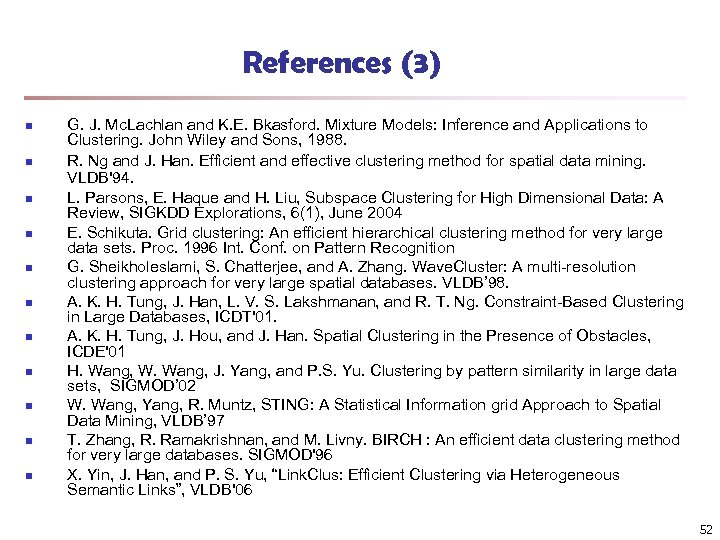References (3) n n n G. J. Mc. Lachlan and K. E. Bkasford. Mixture Models: Inference and Applications to Clustering. John Wiley and Sons, 1988. R. Ng and J. Han. Efficient and effective clustering method for spatial data mining. VLDB'94. L. Parsons, E. Haque and H. Liu, Subspace Clustering for High Dimensional Data: A Review, SIGKDD Explorations, 6(1), June 2004 E. Schikuta. Grid clustering: An efficient hierarchical clustering method for very large data sets. Proc. 1996 Int. Conf. on Pattern Recognition G. Sheikholeslami, S. Chatterjee, and A. Zhang. Wave. Cluster: A multi-resolution clustering approach for very large spatial databases. VLDB’ 98. A. K. H. Tung, J. Han, L. V. S. Lakshmanan, and R. T. Ng. Constraint-Based Clustering in Large Databases, ICDT'01. A. K. H. Tung, J. Hou, and J. Han. Spatial Clustering in the Presence of Obstacles, ICDE'01 H. Wang, W. Wang, J. Yang, and P. S. Yu. Clustering by pattern similarity in large data sets, SIGMOD’ 02 W. Wang, Yang, R. Muntz, STING: A Statistical Information grid Approach to Spatial Data Mining, VLDB’ 97 T. Zhang, R. Ramakrishnan, and M. Livny. BIRCH : An efficient data clustering method for very large databases. SIGMOD'96 X. Yin, J. Han, and P. S. Yu, “Link. Clus: Efficient Clustering via Heterogeneous Semantic Links”, VLDB'06 52